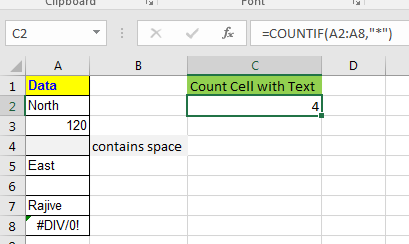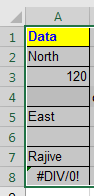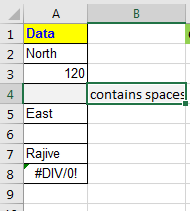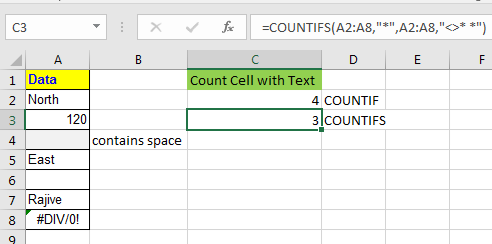# Count the Cells Containing Text

To count the number of cells that have any text (even a single space) we can use COUNTIF function of excel.Generic Formula for Counting Cells containing texts

=COUNTIF(range,”*”)

The wild card character “*” counts any cell that contains text. Let’s see some examples of count cells with text.

COUNTIF Example:
I have data in range A2:A8 (A4 contains space).Now to count the number of cells that have text I will write this COUNTIF formula in cell C2.

=COUNTIF(A2:A8,"*")

And as a result I will get 4.
The will card character “*” only applies to the text, hence the COUNTIF function counts as any cell that contains the text. The numbers and errors arexclueded. (dates are also numbers, hence they will be excluded from the count.)
COUNTIFS Example:
Now sometimes you want to exempt some text to be counted. For our example, we don’t want to count cells who contain spaces only. Here we can use COUNTIFS to count cells containing text with exemption text.

Generic Formula for Counting Cells Containing Text With Exemption Text

=COUNTIFS(range,”*”,range,”text”)

We have the same data. Now we want to count cell containing text only except cells containing spaces only.I am writing this formula in C3:

=COUNTIFS(A2:A8,"*",A2:A8,"<>* *")

We have our count of cells containing any text other than cell containing spaces.How does it work?

The first part A2:A8,"*", looks for any text containing cell.

The second part AA2:A8,"<>* *", excludes any cell containing only spaces.(<> this is NOT operator.)

So yeah, this is the way you can count cells containing text in excel 2016 or older. Let me know if the solution for your problem else explains your problem in the comments section below.

Related Articles:

Count Cells that contain specific text

How to Use COUNT Function in Excel

How to Use COUNTA Function in Excel

Popular Articles:

The VLOOKUP Function in Excel

COUNTIF in Excel 2016

How to Use SUMIF Function in Excel

## Users are saying about us...

1.What formula would you use to count the number of cells in column b that contain text and have adjacent cells in columns c:g that are blank?

Terms and Conditions of use

The applications/code on this site are distributed as is and without warranties or liability. In no event shall the owner of the copyrights, or the authors of the applications/code be liable for any loss of profit, any problems or any damage resulting from the use or evaluation of the applications/code.